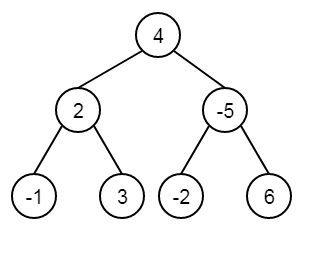# Find maximum level product in Binary Tree in C++

Suppose, one binary tree is given. It has positive and negative nodes. We have to find the maximum product at each level of it.Consider this is the tree, so the product of level 0 is 4, product of level 1 is 2 * -5 = -10, and product of level 2 is -1 * 3 * -2 * 6 = 36. So this is the maximum one.

To solve this, we will perform the level order traversal of the tree, during traversal, process doing the nodes of different levels separately. Then get the maximum product.

## Example

Live Demo

#include<iostream>
#include<queue>
using namespace std;
class Node {
public:
int data;
Node *left, *right;
};
Node* getNode(int data) {
Node* node = new Node;
node->data = data;
node->left = node->right = NULL;
return node;
}
int getMaxLevelProduct(Node* root) {
if (root == NULL)
return 0;
int res = root->data;
queue<Node*> q;
q.push(root);
while (!q.empty()) {
int count = q.size();
int prod = 1;
while (count--) {
Node* temp = q.front();
q.pop();
prod *= temp->data;
if (temp->left != NULL)
q.push(temp->left);
if (temp->right != NULL)
q.push(temp->right);
}
res = max(prod, res);
}
return res;
}
int main() {
Node* root = getNode(4);
root->left = getNode(2);
root->right = getNode(-5);
root->left->left = getNode(-1);
root->left->right = getNode(3);
root->right->left = getNode(-2);
root->right->right = getNode(6);
cout << "Maximum level product is " << getMaxLevelProduct(root) << endl;
}

## Output

Maximum level product is 36

Updated on: 19-Dec-2019

43 Views